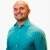# SAT Prep How to determine the area of a base from a word problemThis lesson will look at an example problem involving area. This lesson will give an explanation of how to determine the area of a base from a word problem with a step by step look to... This lesson will look at an example problem involving area. This lesson will give an explanation of how to determine the area of a base from a word problem with a step by step look to better explain the concept.
More... Collapse
5 Views